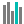#T.DIST (PQL - xl)

This function returns the probability for the t-distribution

• Library: PQL \ Spreadsheet \ Statistical
• Compatibility: Any content (regardless of data source) in the Tabulate spreadsheet module

#### Syntax

T.DIST(x, deg freedom, cumulative)

##### Function Arguments
 Name Description Type Optional x Numeric value used to evaluate the distribution Number deg freedom Number of degrees of freedom to characterize the distribution; if not an integer, the number is truncated Number cumulative A logical value that determines the form of the function. If cumulative is TRUE, the function returns the cumulative distribution function; if FALSE, it returns the probability density function StringOrNumber